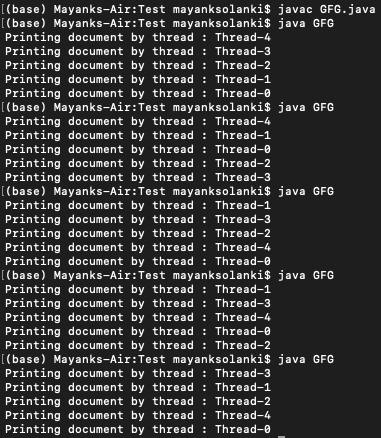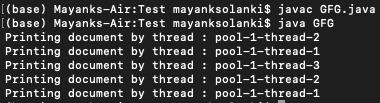Open in App
Not now

# FixedSizeThreadPoolExecutor in Java Executor Framework

• Last Updated : 05 Jul, 2021

In a fixed thread pool, the number of threads in the pool depends on the type of task. For CPUs-intensive tasks like encryption, implementing hash algorithms, the number of threads depends on the number of cores. Thus, every thread runs on its core and once it is done executing the task it picks up the next task from the queue. Also, there could be a possibility that multiple applications are running, so not all cores might be accessible.

For an IO-intensive task that involves too many DB calls or HTTP calls, if there are fewer threads, then there might be too many threads waiting for these IO calls to complete. Thus, CPUs time is wasted and so it makes sense to have more threads. In this way, few threads get to do some processing/calculations utilizing the CPU time and few threads might be waiting for DB/HTTP calls to complete.

Approach 1: Standard approach

In java, when you want to run your task in an asynchronous manner, you spawn threads and make the threads execute the task. For this, we have a class that implements the Runnable interface. Then we pass an instance of Runnable to the thread which then executes the task.

Example

## Java

 `// Java Program to Test Threads` `// Importing required packages``import` `java.io.*;``import` `java.util.*;` `// Main class``// to test the threads``public` `class` `GFG {` `    ``// Main driver method``    ``public` `static` `void` `main(String[] args)``    ``{` `        ``// Creating 5 threads and passing an instance``        ``// of Runnable implementation``        ``for` `(``int` `i = ``0``; i < ``5``; i++) {` `            ``// Creating object of Thread class``            ``Thread thread = ``new` `Thread(() -> {``                ``// Printing and display the current thread``                ``// using curentThread() and getName() method``                ``System.out.println(``                    ``"Printing document by thread : "``                    ``+ Thread.currentThread().getName());` `                ``// Try block to check for exceptions``                ``try` `{` `                    ``// Making current threads to sleep``                    ``// for 1 second``                    ``Thread.sleep(1000L);``                ``}` `                ``// Catch block to handle th exceptions``                ``catch` `(InterruptedException e) {` `                    ``// Print the line number where exception``                    ``// occurred``                    ``e.printStackTrace();``                ``}``            ``});` `            ``// Starting the threads using start() method``            ``thread.start();``        ``}``    ``}``}`

Note: The order of executing the task changes with each run.Output Explanation:

Here in the main thread spawns 5 threads, each of which given an instance of Runnable implementation. When we say thread.start(), the runnable implementation’s run method gets invoked and the task is executed.

Approach 2:

Example:

## Java

 `// Java Program to demonstrate FixedThreadPoolExecutor` `// Importing required libraries``import` `java.util.concurrent.ExecutorService;``import` `java.util.concurrent.Executors;` `// Main class``public` `class` `GFG {` `    ``// Main driver method``    ``public` `static` `void` `main(String[] args)``    ``{` `        ``// Creating an object of ExecutorService for``        ``// asking the executor service to create a thread``        ``// pool with fixed number of threads``        ``ExecutorService service``            ``= Executors.newFixedThreadPool(``3``);` `        ``// Creating 5 threads using loops``        ``for` `(``int` `i = ``0``; i < ``5``; i++) {` `            ``// Submitting task to service's execute method``            ``service.execute(() -> {``                ``// Printing and display the current thread``                ``// using curentThread() and getName() method``                ``System.out.println(``                    ``"Printing document by thread : "``                    ``+ Thread.currentThread().getName());` `                ``// Try block to check for exceptions``                ``try` `{` `                    ``// Making threads to sleep for 1 second``                    ``// using the sleep() method``                    ``Thread.sleep(1000L);``                ``}` `                ``// Catch block to handle the exceptions``                ``catch` `(InterruptedException e) {` `                    ``// Print and display the line number``                    ``// where the exception occurred``                    ``e.printStackTrace();``                ``}``            ``});``        ``}` `        ``// In order to avoid further coming execution of``        ``// tasks shutdown() method is used``        ``service.shutdown();``    ``}``}`

Output:Output Explanation:

Here we created a thread pool with 3 threads where we submitted 5 tasks to the executor service. This executor service takes care of executing the submitted task by the threads from the thread pool. The executor service takes care of creating the thread pool. So here thread name is pool-X-thread-Y.

My Personal Notes arrow_drop_up